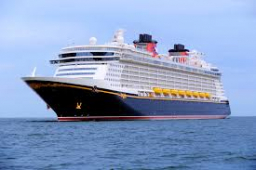# The distance 2

The distance between two ports M and N is 2100km. If two ships travel towards each other with one ship leaving port M at 20km/h and at the same time another ship from port N travel 15km/h.

(I) How long will it take the two ships to meet.
(ii) how far will they be from port M when they meet

t =  60 h
s1 =  1200 km

### Step-by-step explanation:Did you find an error or inaccuracy? Feel free to write us. Thank you!

Tips for related online calculators
Need help calculating sum, simplifying, or multiplying fractions? Try our fraction calculator.
Do you have a linear equation or system of equations and looking for its solution? Or do you have a quadratic equation?
Do you want to convert length units?
Do you want to convert velocity (speed) units?
Do you want to convert time units like minutes to seconds?